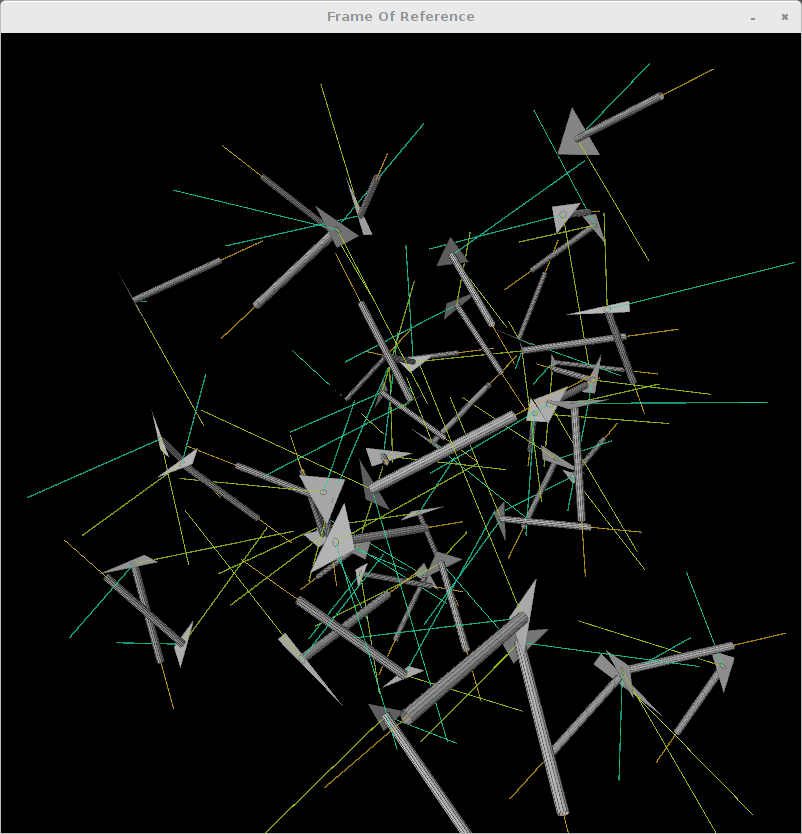Here is vanilla processing sketch by Ira Greenberg translated for JRubyArt. This sketch features the use of MDMatrix (from the mdarray gem) to do 3D transforms. The mdarray gem was inspired by numpy and it provides a similar interface. However in this example and possibly others, performance is dreadful compared to using a regular ruby matrix. Also featured are Vec3D (replaces PVector), ArcBall (for intuitive manipulation) and AppRender for efficient Vec3D to vertex translation.

###frame_of_reference.rb

``````###############
# Frame of Reference example by Ira Greenberg
# https://github.com/irajgreenberg/ProcessingTips
# Translated to JRubyArt by Martin Prout February 2016
###############

FACE_COUNT = 50

def settings
size(800, 800, P3D)
end

def setup
sketch_title 'Frame Of Reference'
ArcBall.init(self) # so we use mouse to rotate sketch and mouse wheel to zoom
@c = []
@p = []
FACE_COUNT.times do |i|
# calc some random triangles in 3 space
val = Vec3D.new(
rand(-width / 2..width / 2),
rand(-width / 2..width / 2),
rand(-width / 2..width / 2)
)
v0 = Vec3D.new(
rand(-val.x..-val.x + 100),
rand(-val.y..-val.y + 100),
rand(-val.z..-val.z + 100)
)
v1 = Vec3D.new(
rand(-val.x..-val.x + 100),
rand(-val.y..-val.y + 100),
rand(-val.z..-val.z + 100)
)
v2 = Vec3D.new(
rand(-val.x..-val.x + 100),
rand(-val.y..-val.y + 100),
rand(-val.z..-val.z + 100)
)
p << Plane.new([v0, v1, v2])
# build some cute little cylinders
c << Cylinder.new(Vec3D.new(150, 5, 5), 12)
# Using each Triangle normal (N),
# One of the Triangle's edges as a tangent (T)
# Calculate a bi-normal (B) using the cross-product between each N and T
# Note caps represent constants in ruby so we used N = nn, T = tt and
# B = bb in the ruby code below

# A picture helps
# nice, sweet orthogonal axes

# N   B
# |  /
# | /
# |/____T

#
# N, T, B together give you a Frame of Reference (cute little local
# coordinate system), based on each triangle. You can then take the
# cylinder (or any vertices) and transform them using a 3 x 3 matrix to
# this coordinate system. (In the matrix each column is based on N, T and
# B respecivley.) The transform will handle any rotations and scaling, but
# not the translation, but we can add another dimenson to the matrix to
# hold the translation values. Here's what all this confusing description
# looks like:

#
# Matrix :                               Vector :
# |  N.x  T.x  B.x  translation.x  |      |  x  |
# |  N.y  T.y  B.y  translation.y  |      |  y  |
# |  N.z  T.z  B.z  translation.z  |      |  z  |
# |  0    0    0    1              |      |  1  |

# We add the extra row in the matrix and the 1 to each vector
# so the math works. We describe the Matrix as 4 rows by 4 columns
# and the vector now as a Matrix with 4 rows and 1 column.
# When you multiply matrices the inner numbers MUST match, so:
# [4 x 4] [4 x 1] is OK, but [4 x 4] [1 x 4] is NOT COOL.
# see mat4.rb where we use MDMatrix to handle the multiplication for us

nn = p[i].n
tt = Vec3D.new(
p[i].vecs.x - p[i].vecs.x,
p[i].vecs.y - p[i].vecs.y,
p[i].vecs.z - p[i].vecs.z
)
nn.normalize!
tt.normalize!
bb = nn.cross(tt)
# build matrix with frame and translation (to centroid of each triangle)
m4 = Mat4.new(xaxis: nn, yaxis: tt, zaxis: bb, translate: p[i].c)
# transform each cylinder to align with each triangle
c[i].vecs = m4 * c[i].vecs
end
fill(187)
stroke(50, 20)
end

def draw
background(0)
lights
FACE_COUNT.times do |i|
p.each(&:display)
c.each(&:display)
end
end

def renderer
@renderer ||= AppRender.new(self)
end
``````

### cylinder.rb

``````# encoding: UTF-8
# frozen_string_literal: true
# Cylinder class can access sketch methods thanks to Processing::Proxy module
class Cylinder
include Processing::Proxy
attr_accessor :vecs

def initialize(dim, detail)
@dim = dim
@detail = detail
init
end

def init
#    created around x-axis
#    y = Math.cos
#    z = Math.sin
veca = []
vecb = []
(0...360).step(360 / detail) do |theta|
cost = DegLut.cos(theta)
sint = DegLut.sin(theta)
veca << Vec3D.new(0, cost * dim.y, sint * dim.z)
vecb << Vec3D.new(dim.x, cost * dim.y, sint * dim.z)
end
@vecs = veca.concat(vecb)
end

def display
detail.times do |i|
vecs[i].to_vertex(renderer)
if i < (detail - 1)
vecs[i + 1].to_vertex(renderer)
vecs[detail + i + 1].to_vertex(renderer)
else
vecs.to_vertex(renderer)
vecs[detail].to_vertex(renderer)
end
vecs[detail + i].to_vertex(renderer)
end
end_shape
end
end
``````

### plane.rb

``````# encoding: UTF-8
# frozen_string_literal: true
NORM_LEN = 225.0
# Plane class can access sketch methods thanks to Processing::Proxy module
class Plane
include Processing::Proxy

def initialize(vecs)
@vecs = vecs
init
end

def init
v1 = vecs - vecs
v2 = vecs - vecs

@c = Vec3D.new(
(vecs.x + vecs.x + vecs.x) / 3,
(vecs.y + vecs.y + vecs.y) / 3,
(vecs.z + vecs.z + vecs.z) / 3
)

@n = v1.cross(v2)
n.normalize!
end

def display

begin_shape(TRIANGLES)
vecs.map { |vec| vec.to_vertex(renderer) }
end_shape

# normal
stroke(200, 160, 30)
begin_shape(LINES)
c.to_vertex(renderer)
(c + n * NORM_LEN).to_vertex(renderer)
end_shape

# binormal
stroke(160, 200, 30)
begin_shape(LINES)
c.to_vertex(renderer)
# tangent
v = vecs.copy
v -= vecs
v.normalize!
(c + v * NORM_LEN).to_vertex(renderer)
end_shape

stroke(30, 200, 160)
begin_shape(LINES)
c.to_vertex(renderer)
b = v.cross(n)
(c + b * NORM_LEN).to_vertex(renderer)
end_shape
stroke(0, 75)
end
end
``````

### mat4.rb

``````# uber simple Homogeneous 4 x 4 matrix
require 'mdarray'

class Mat4

def initialize(xaxis:, yaxis:, zaxis:, translate:)
@mat = MDMatrix.double([4, 4], [
xaxis.x, yaxis.x, zaxis.x, translate.x,
xaxis.y, yaxis.y, zaxis.y, translate.y,
xaxis.z, yaxis.z, zaxis.z, translate.z,
0, 0, 0, 1]
)
end

# The processing version changes the input 'array', here we return
# a new array with transformed values (which we then assign to the input)
# see line 89 Frame_of_Reference.rb, NB: regular ruby Matrix is much faster

def *(other)
other.map.each do |arr|
matrix_to_vector(mat * MDMatrix.double([4, 1], [arr.x, arr.y, arr.z, 1]))
end
end

private

# It isn't obvious but we only require first 3 elements
def matrix_to_vector(vec)
Vec3D.new(vec[0, 0], vec[1, 0], vec[2, 0])
end
end
``````

### snapshot of the running sketch